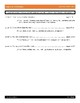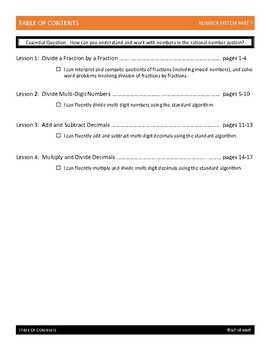# 6th Grade Number System (Part I) Unit (Bundle)Subject
Resource Type
File Type

PDF

(7 MB|36 pages)
Product Rating
4.0
(1 Rating)
Standards
• Product Description
• StandardsNEW
Sixth Grade Number System Unit. This bundle includes 4 number system lessons. Each lesson contains guided notes, guided practice, independent practice (HW), and answer keys.

Lessons include:
1 - Divide a Fraction by a Fraction
2 - Divide Multi-Digit Numbers
3 - Add and Subtract Decimals
4 - Multiply and Divide Decimals

The complete SIXTH GRADE MATH BUNDLE can be viewed or downloaded by clicking here : This bundle includes all 46 of my lessons at a 40% savings when compared to buying each lesson individually.

Credits:
Cover border by Miss Giraffe
Specialty fonts (on cover page) by Kimberly Geswein Fonts
Fluently add, subtract, multiply, and divide multi-digit decimals using the standard algorithm for each operation.
Fluently divide multi-digit numbers using the standard algorithm.
Interpret and compute quotients of fractions, and solve word problems involving division of fractions by fractions, e.g., by using visual fraction models and equations to represent the problem. For example, create a story context for (2/3) ÷ (3/4) and use a visual fraction model to show the quotient; use the relationship between multiplication and division to explain that (2/3) ÷ (3/4) = 8/9 because 3/4 of 8/9 is 2/3. (In general, (𝘢/𝘣) ÷ (𝘤/𝘥) = 𝘢𝘥/𝘣𝘤.) How much chocolate will each person get if 3 people share 1/2 lb of chocolate equally? How many 3/4-cup servings are in 2/3 of a cup of yogurt? How wide is a rectangular strip of land with length 3/4 mi and area 1/2 square mi?
Total Pages
36 pages
Included
Teaching Duration
1 Week
Report this Resource to TpT
Reported resources will be reviewed by our team. Report this resource to let us know if this resource violates TpT’s content guidelines.